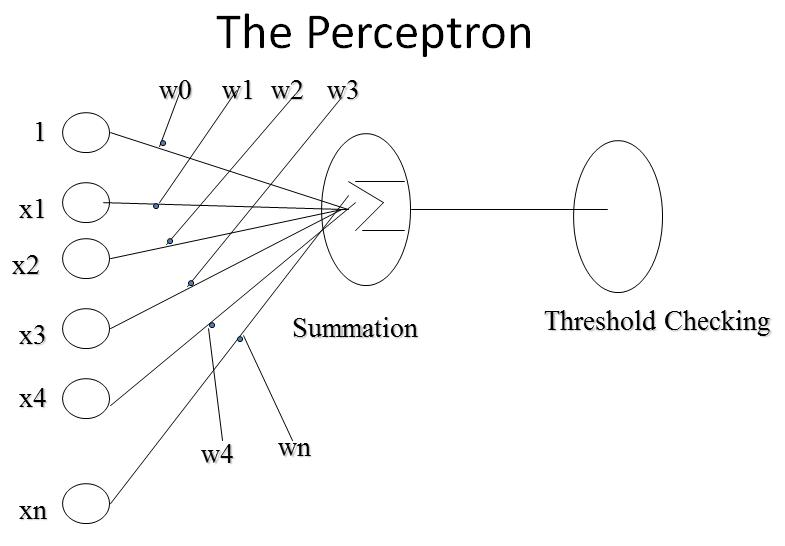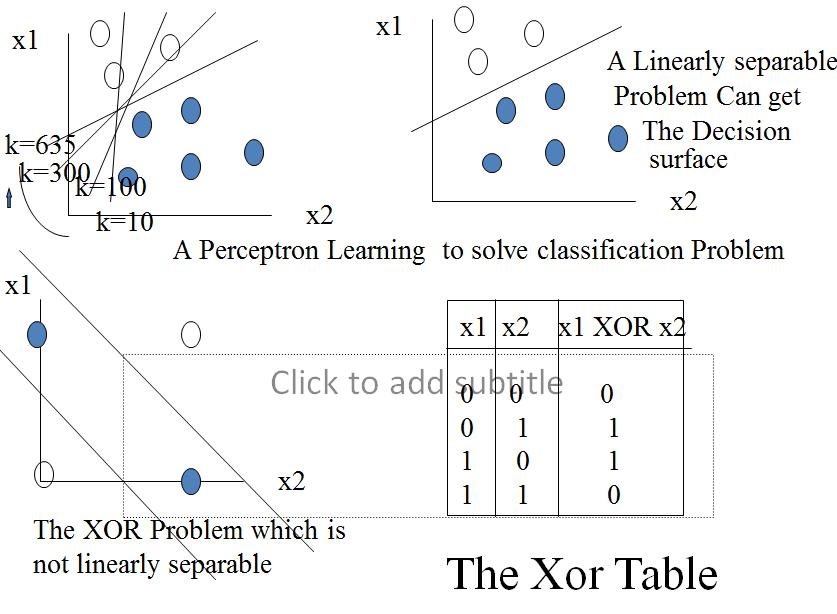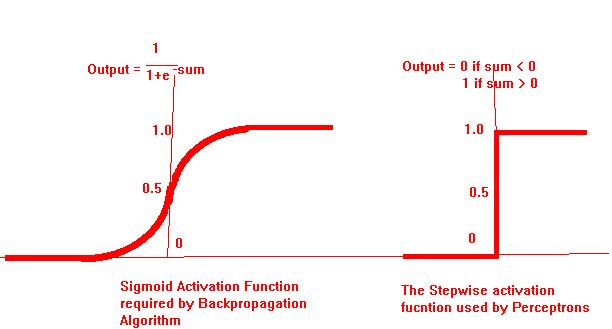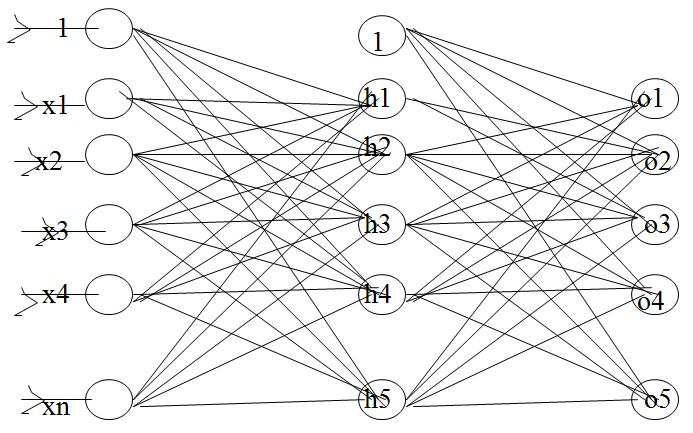Search:

Submit Article

Perceptron in Neural Networks

Posted By: Shruti Sharma     Category: Artificial Intelligence     Views: 17636

Perceptron in Neural Networks

Perceptron

Like the brain neuron, it is bi-stated.
fires when input summation going above threshold value
Given input-output pairs, can make itself  learn through changing weights.
Used as a building block in Different brand of current NNs even though not used nowLearning of Perceptron
• Random initialization of weights
• Patterns are applied one by one.
• Positively misclassified input, weights decreased in proportion with the input
• Negatively misclassified inputs, weights are decreased by the same proportion.
• When all patterns are over, the whole set is reapplied until no wrong answer is got.
• For a linearly separable problem, Perceptron can always solve the problem, Perceptron Convergence theorem of Rosenblatt says.
• The learning of Perceptron is analogous to adjusting water tap containing cold and hot water inputs

How Perceptron computes
• Let Input is a vector (x1,x2,..xn)
• x0 = 1 (to have the effect of threshold)
• g(x) = S n i=0 (wi * xi) for i=0 to n
• o(x) = 0 if g (x) < 0 else 1
• If  two inputs then g(x) = w0+w1x1+w2x2
• When g(x) is 0, w2 = -(w1/w2)*x1- w0/w2
• This is an equation of decision surface
• Sign of g(x) determines whether Perceptron fires or not, while magnitude ( the value of g(x)) determines how far it is from the decision surface.
• Let w be the set of weights (w0,w1,...wn)
• let X be the subset of training instances misclassified by the current set of weights
• J(w) is the Perceptron Criterion function
• J(w) = S(S(wi*xi)for i=0 to n) for all x belongs to X
• J(w) = E(|wx|) for all x belongs to X
• J(w) = E w * x if x is misclassified as a negative example otherwise w*-x if x is misclassified as a positive example.
• Let d(J) = S(x) where x is taken positive if x misclassified as a negative example, otherwise x is taken negative.
• dis  called a gradient which is telling us the direction in which we should move to reduce J, i. e. the direction of solution
• New set of weights is determined as follows
•     w(t+1) = w(t) + h * d(J)

Perceptron Learning Algorithm
• Given  a Classification problem with n input features and 2 output classes
• The solution sought is the one out of potentially infinite number of weight vectors that will cause the Perceptron to fire whenever the input falls into the first output class
• n+1 inputs and n+1 weights are taken, where x0 always set to 1
• All weights are initialized to different random values.
• Iterate through The training set, collecting all inputs misclassified by the current set of weights.
• If all inputs are classified correctly, output the weights and quit.
• Otherwise compute the vector sum S of the misclassified input vectors, where each vector has the form (x0,x1,..xn). In Creating the sum add S to vector x if x is an input for which the Perceptron incorrectly fails to fire, but add -x if x is an input for which Perceptron incorrectly fires. Multiply the sum by scale factor h
• Modify the weights (w0..wn) by adding the elements of the vector S to them. Go to Iteration step.

Analysis of the algorithm :-

- It is a search process of its kind. It starts from a state where all weights are random and stops when get the weights which constitutes the solution
- The decision surface is a line here. There can be infinite equations of a line so infinite no. of weight vectors possible to get. This is also true for other cases

Problem with Perceptron learning algorithm
• Answer can’t be sought when no decision surface exists. i.e for EXOR problem.
• Multilayer Perceptrons can solve problem by creating multiple decision surfaces.
• But it was not known how to train middle layer weights. With fixed handcoded weights , though problem could be solved.
• Perceptron Convergence theorem could not be extended to Multilayer networksMultilayer Perceptrons and Backpropagation
• Backpropagation is a systematic method of training multilayer Perceptrons
• It was first shown by Werbos in 1974, then Parker doing the same in 1982 and the same is rediscovered by Rumelhart and others in 1986.
• Despite of limitations Backpropagation has dramatically expanded the range of problems which could be solved thru NN
• Backpropagation uses an algorithm which has strong mathematical background.
• It requires an activation function which is everywhere differentiable.
• It is using a sigmoid type Activation function compared to a squared Activation function by single layer Perceptron.
• Sigmoid allows automatic gain control and also it is useful in bettering a trained weight.

Comparison of Activation FunctionsMulti-layer PerceptronMultilayer network
• Multilayer Network Shown earlier is trained by Backpropagation algorithm.
• First layer is known as input, Second is known as hidden and the third is known as output layer.
• The first layer just distributes the inputs, other layers sums and applies the sigmoid function to their respective inputs.
• There are two layers of weights.
• There is inconsistency in literature about the layers in the Network.
Share:

Didn't find what you were looking for? Find more on Perceptron in Neural Networks Or get search suggestion and latest updates.Shruti Sharma author of Perceptron in Neural Networks is from United States. Shruti Sharma says Hello Everyone,I am Shruti Sharma from United States View All Articles